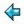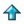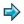### matrix.m

```|| very simple matrix package (DT)
|| to include this in one of your own scripts, say
||      %include <ex/matrix>

%export matrix idmat matadd matsub matmult scalmult mkrow mkcol det

matrix == [[num]]

idmat :: num->matrix ||identity matrix of given size
idmat n = [[delta i j|j<-[1..n]]|i<-[1..n]]
where
delta i j = 1, if i=j
= 0, otherwise

where
vadd x y = map2 (+) x y

matsub :: matrix->matrix->matrix
matsub x y = map2 vsub x y
where
vsub = map2 (-)

matmult :: matrix->matrix->matrix
matmult x y = outer inner x (transpose y)  ||*
inner x y = sum (map2 (*) x y)
outer f x y = [[f a b|b<-y]|a<-x]

||*note that transpose is already defined in the standard environment

scalmult :: num->matrix->matrix ||multiply a matrix by a scalar
scalmult n x = map (map (*n)) x

mkrow :: [num]->matrix ||make vector into matrix with a single row
mkrow x = [x]

mkcol :: [num]->matrix ||make vector into matrix with a single column
mkcol x = map (:[]) x

det :: matrix->num ||determinant, of square matrix
det [[a]] = a
det xs = sum [xs!0!i*cofactor 0 i xs|i<-index xs], if #xs=#xs!0
= error "det of nonsquare matrix",          otherwise
cofactor i j xs = (-1)^(i+j) * det (minor i j xs)
minor i j xs = [omit j x | x<-omit i xs]
omit i x = take i x ++ drop (i+1) x

adjoint xs = transpose[[cofactor i j xs | j<-index xs] | i <- index xs]

inv :: matrix->matrix ||inverse, of non-singular square matrix
inv xs = transpose[[cofactor i j xs/h | j<-index xs] | i <- index xs]
where
h = det xs
||The above is a literal transcription of the mathematical definition of
||matrix  inverse.   A  less  naive version of the package would rewrite
||this to use Gaussian elimination.

||a few test matrices (these are not exported from the script, but  will
||be in scope if this is your current script)
a = [[1,2],[3,4]]
b = [[1,1,1],[1,2,3],[2,4,8]]
c = [[0,1,2,3],[1,2,3,4],[2,3,4,0],[3,4,0,1]]
i2 = idmat 2
i3 = idmat 3
i4 = idmat 4

test = matmult b (inv b)
```